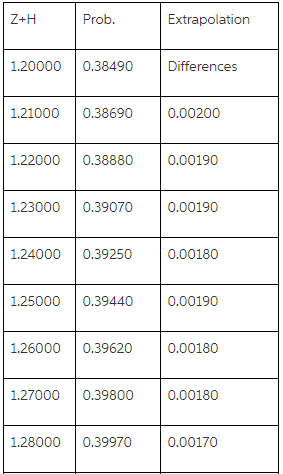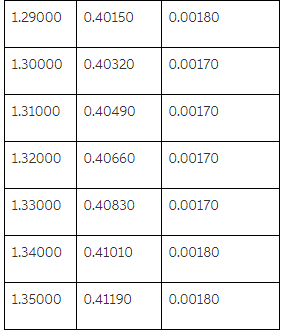# Use the following Normal Distribution table to calculate the area under the Normal Curve

Use the following Normal Distribution table to calculate the area under the Normal Curve (Shaded area in the Figure) when Z=1.3 & H=0.05; Assume that you do not have vales of the area beyond z=1.2 in the table; i.e. you may need to use the extrapolation. Check your calculated value and compare with the values in the table [for z=1.3 & H=0.05]. Calculate your percentage of error in the estimation.

How do I solve this problem using extrapolation?

Extrapolation:

Use the following Normal Distribution table to calculate the area under the Normal Curve (Shaded area in the Figure) when Z=1.3& H=0.05; Assume that you do not have vales of the area beyond z=1.2 in the table; i.e. you may need to use the extrapolation. Check your calculated value and compare with the values in the table [for z=1.3 & H=0.05]. Calculate your percentage of error in the estimation.

How do I solve this problem using extrapolation?

Solution:

Here, we have to use the technique of extrapolation for finding the probability for the z = 1.35 (Z=1.3& H = 0.05). Let us see this discuss this question step by step given as below:

For using extrapolation we need the values for the row of z = 1.2
The values for the row z and columns H are given as below:
By using the extrapolation, we getWe get P(Z<1.35) = 0.4119 by using the extrapolation however the probability by using the table is given as 0.4115. This means there is a very small difference observed due extrapolation.